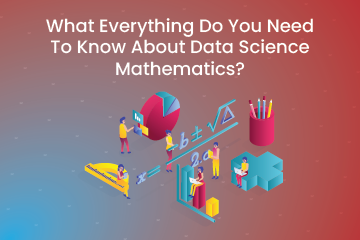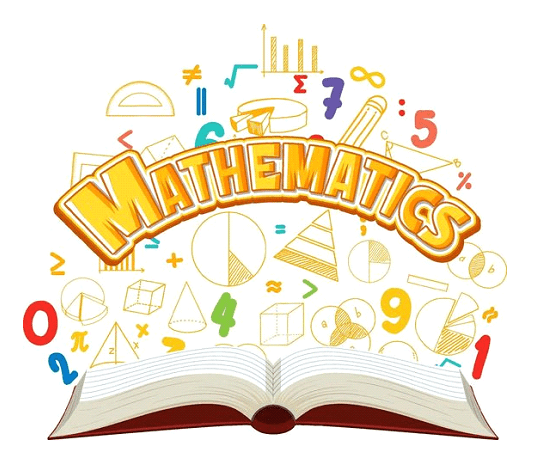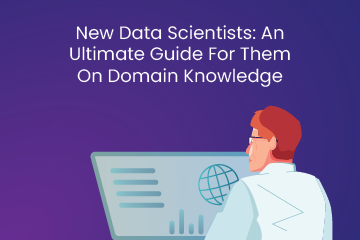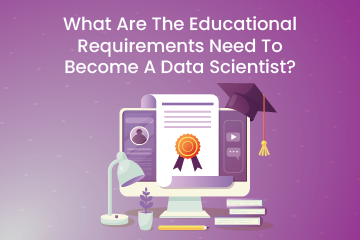Home / Blog / Data Science / What Everything Do You Need To Know About Data Science Mathematics?

# What Everything Do You Need To Know About Data Science Mathematics?

• February 23, 2023
• 2894
• 95### Meet the Author : Mr. Bharani Kumar

Bharani Kumar Depuru is a well known IT personality from Hyderabad. He is the Founder and Director of Innodatatics Pvt Ltd and 360DigiTMG. Bharani Kumar is an IIT and ISB alumni with more than 17 years of experience, he held prominent positions in the IT elites like HSBC, ITC Infotech, Infosys, and Deloitte. He is a prevalent IT consultant specializing in Industrial Revolution 4.0 implementation, Data Analytics practice setup, Artificial Intelligence, Big Data Analytics, Industrial IoT, Business Intelligence and Business Management. Bharani Kumar is also the chief trainer at 360DigiTMG with more than Ten years of experience and has been making the IT transition journey easy for his students. 360DigiTMG is at the forefront of delivering quality education, thereby bridging the gap between academia and industry.

Mathematical knowledge is necessary for data science careers because it depends on machine learning algorithms, data analysis, and insight discovery. Although there are other requirements for your degree and employment in data science, math is frequently one of the most crucial. For example, one of the essential elements of a data scientist's workflow is locating and comprehending business difficulties before transforming them into quantitative ones.Our everyday lives depend significantly on mathematics. Right from the moment we are born until we pass away, we employ mathematics, consciously or unconsciously, in many situations. As children, we start studying numbers, tables, equations, etc., working our way up to solving complex problems, creating new formulae, and developing novel methods and strategies for a specific mathematical issue.

Mathematics has grown in importance in cutting-edge technologies like Machine Learning, Artificial Intelligence, Data Science, Deep Learning, and many others. Mathematics is directly or indirectly tied to every new technology in today's society to create clever and straightforward solutions to challenges. Through its applications in numerous sectors, mathematics is currently dominating the world like a king. With its various math functions, novel methodologies, theorems, algorithms, and other workplace applications, mathematics is helpful in many industries, including software, medical, automotive, designing, robotics, and many more.,

### Learn the core concepts of Data Science Course video on YouTube:

Looking forward to becoming a Data Scientist? Check out the Data Science Course and get certified today.

### Important Mathematical Concepts for Data Science and Machine Learning:

You might not need to grasp math if your background is more in engineering (building & managing data infrastructures, designing ETL pipelines). On the other hand, suppose you want to grasp machine learning in general and deep learning in particular. Then, you should get familiar with mathematical topics like probability theory and linear algebra.

1. Probability and Statistics: Data preprocessing, feature transformation, imputation, dimensionality reduction, feature engineering, model evaluation, etc., all employ statistics and probability.

2. Multivariable Calculus: Many features or predictors are present in the datasets used to build most machine learning models. Therefore, multivariable calculus knowledge is crucial for creating a machine-learning model.

3. Linear Algebra:Most crucial mathematical ability in machine learning is the linear algebra. One uses a matrix to represent a data set. Data preprocessing, transformation, dimensionality reduction, and model evaluation all involve linear algebra.

4. Optimization Techniques:Most machine learning algorithms learn the weights that should be applied to the testing data to generate the predicted labels by minimizing an objective function.Want to learn more about data science? Enroll in the Best Data Science courses in Chennai to do so.

### The Ideal Method for Learning Math for Data Science is:

Unfortunately, the self-starter method is to "do crap" to learn math for data science. Instead, we will approach linear algebra and calculus by applying them to actual algorithms!

However, it would help if you had the first study or reviewed the underlying theory. Even though you don't have to read the entire textbook, you should first understand the essential ideas.

The three steps to mastering the arithmetic needed for data science and machine learning are as follows:

• Matrix algebra and eigenvalues get covered in Linear Algebra for Data Science.
• Calculus for Data Science: gradients and derivatives
• Implement a primary neural network using gradient descent starting from scratch.

1) Data Science Linear Algebra: Linear algebra is a crucial component of many machine-learning ideas. For instance, matrix multiplication is necessary for both regression and PCA, respectively. However, most ML applications also work with high-dimensional data (data with many variables). Matrix representations work best for this kind of data.

2) Data Science Calculus: Many crucial ML applications require knowledge of calculus—an illustration. For example, you must be able to compute derivatives and gradients for optimization. One of the most commonly used optimization methods is the Gradient descent.

3) Create a Simple Neural Network from Scratch: Creating a straightforward neural network from scratch is one of the finest ways to study math for data science and machine learning. One will represent the network using linear algebra and optimize it using calculus. You will specifically write new code for gradient descent.

### What Connection Does Math Have to Modern Technology?

We've discussed how mathematics is crucial to developing contemporary technologies like machine learning, artificial intelligence, data science, deep learning, etc. Each algorithm used to create modern technologies has a mathematical purpose. Likewise, every issue we run through in our daily applications is logically explained by mathematics.

### 4 Major Mathematics Subjects Needed to Become a Data Scientist:

Most data analysts only employ a few minor subsections of mathematics daily, depending on the field (and occasionally the project). All data analysts should be familiar with linear algebra, probability, statistics, and calculus, but not all careers or jobs necessitate a deep understanding of these or other advanced subjects.

While mastering more complex math concepts (such as calculus and beyond) expands your toolkit and teaches you how to solve problems, it can also be a roadblock that prevents you from moving forward on your journey to becoming a data scientist.

### 1. Linear Algebra:

It is a field of mathematics that focuses on finding solutions to linear equations with unknown values. It also serves as the theoretical foundation for machine learning.

While machine learning may not fall under the purview of a general data analyst's day-to-day tasks, data pretreatment and data transformation involve the application of linear algebra's core ideas. Furthermore, learning linear algebra teaches you how to reason logically through a series of steps, which is helpful when conducting an analysis that focuses on providing an answer to a particular issue or resolving a specific problem.

Vectors, spaces, matrix transformations, and different coordinate systems are all concepts in linear algebra. In data analysis, you can use vectors to determine how dissimilar a prediction from a data collection is from the expected result following data transformation. During data transformation, you employ matrix transformations to change one vector into another to represent data geometrically in a two-dimensional or three-dimensional space. Finally, you can use alternative coordinate systems to alter the visual representation of datasets in data analysis, ensuring that the data is properly represented.

Also, check this Data Science Institute in Bangalore to start a career in Data Science.

### 2. Probability:

Probability is the study of how likely something is to occur and is crucial for forming judgments that can assist in making decisions in ambiguous circumstances. However, despite their connection and frequent combination in studies, probability and statistics are employed to get distinct findings.

Finding the possibility that a recession will happen, the likelihood that you connect an illness to the frequency of a gene, or even the opportunity that a visitor to a website will sign up for its newsletter are few of the several practical applications of probability in various industries.

You can utilize two different probabilities to examine data sets. The kind of probability with associated rules is known as classical probability. For instance, you may establish a requirement that a website's likelihood of generating sales from visitors must be greater than 0.33. Relative frequency is a type of probability that examines the ratio of the occurrence of one event to all other potential outcomes. You can use it to compare the development of a subset of data to the overall amount of data gathered.

### 3. Calculus:

This area of mathematics focuses on the analysis of ongoing changes and the final optimization of outcomes. It is challenging to compute the probabilities, and we must develop better solutions to the difficulties with solid calculus understanding. It emphasizes limits, integrals, and derivatives.

Become a Data Scientist with 360DigiTMG Data Science course in Hyderabad Get trained by the alumni from IIT, IIM, and ISB.

### 4. Statistics:

They enable us to understand the facts. It focuses on gathering, showing, analyzing, and interpreting numerical data. An organization's development and progress are primarily attributed to statistics, a fundamental component of the newest technologies that allow the analysis of increasingly complex data.

Inferential Statistics and Descriptive Statistics make up the majority of this classification. The notion is commonly employed in mathematics and has a higher significance in generating new types of applications and algorithms that contribute to advancing new technologies. Statistics enable us to provide a broad overview of how a specific sector of the economy or a particular workplace operates. What role does mathematics play as the foundation of computer science and statistics?

Let's begin with statistics, the study of speculating. As a result, it serves as the foundation for artificial intelligence and machine learning. Statistics applies numerous mathematical techniques to get a result. For instance, understanding the operation of a probability density function will enable us to choose the appropriate distribution for a given problem statement. To create a curve replicating our data's distribution, we need to understand how that function integrates when we look at the data. It is only one of many straightforward instances; there are many more areas of statistics where mathematical theory is necessary to bridge the gap between data and educated guesses.

Let's similarly talk about computer science. Let me focus on the two main areas of computer science that have the biggest influence on data science: databases and the use of a programming language, like Python, for any machine learning work. Both cases go through a lot of matrix operations. With an understanding of linear algebra, you might use methods for performing database operations or any machine learning task implementation, but they would be more effective. Here, one will use the skills of database performance engineers and machine learning experts. With appropriate cost and resource allocation, they optimize and deploy.### Situations in Everyday Life that need an Understanding of Fundamental Mathematics:

You could counter that since the libraries already abstract all these ideas, why not build directly on top of them? Let's examine some real-world situations that an aspirant machine/deep learning practitioner would encounter daily to fight that.

• Choosing models based on the constraints they have by nature:

Because of its scalability and computing complexity, a model is frequently not employed in production (the actual product) despite performing quite well. Even if it is not the best model, understanding the underlying limits of your training process might help you select the appropriate model for your use case.

What we know about the cost functions is an excellent place to start. For instance, a linear regression model's Mean Square Error (MSE) cost function is a convex function. Since the slope of this continuous convex function never changes abruptly, we must utilize gradient descent since it ensures that we will eventually arrive arbitrarily close to the global minimum, which is the objective of the cost function.

• Using machine learning to address problems in particular domains:

Any product-based company would expect the data scientist to use his analysis and model results to support critical decisions; thus, they need to be well-versed in the subject area, whether bioinformatics, banking, e-commerce, or disease diagnosis. In addition, finance, banking, and other computationally intensive fields call on a solid mathematical foundation.

For instance, a data scientist who works as a quant in a hedge fund and is creating a model to price derivative securities should know how calculus, normal distribution, and log returns influence their model's development.

The research-oriented, multi-billion dollar areas, including drug discovery, still largely rely on traditional statistical analysis, which calls for an understanding of statistical concepts like mean, standard deviation, sampling, bootstrapping, kurtosis, skewness, etc.

• Creating effective Learning systems:

As a data scientist, you must be familiar with machine learning methods and use that knowledge to create more effective models. Choosing the performance measure for your issue is a nice basic illustration of this; a performance measure indicates how much inaccuracy your system produces when predicting.

Using the regression problem of predicting home prices with a dataset full of outliers as an example, Even though outliers would increase the size of the errors as a result of the squared term in the formula, someone who only understands that Root Mean Square Error (RMSE) is the chosen performance indicator for regression models will base their evaluation of the model on that.

• Understanding and debugging machine learning (ML) algorithms:

Debugging a software program is simple since there are only two things to consider: the algorithm or its implementation. Developing intuition about potential problem locations is simpler, but debugging machine learning becomes quite challenging due to the additional dimensions introduced by the data and model of choice. As a result, your algorithm either needs to be fixed or work better.

Fortunately, we have further clues to identify the bug's location. A person with a solid understanding of multivariate calculus would have a clearer understanding of how the gradient descent's cost function is optimized. Knowing math will make it easier for you to test your learning systems (models)

• Job Interviews:

In addition to being familiar with Sci-kit Learn and Tensorflow, candidates for the position of data scientist must also understand how a decision tree determines the impurity at each node, how a linear regression model's cost function is optimized, and what the decision function for a linear SVM classifier is.

### Wrap-up:

Data science has developed into a synthesis of several fields, including computer science, statistics, business acumen, and communication prowess, with mathematics at its core. Moreover, mathematics as a topic can help build logical thinking and the attitude to consider several choices to find a solution if you study it with the appropriate philosophy.

If you truly want to pursue a career in data science, you should, at the very least, be familiar with concepts like linear algebra, which deals with vectors and matrices, probability distribution, which you use to measure uncertainty, scalars, and vector calculus, which explains gradient descent. To get an idea, enroll in 360digiTMG now!

### Data Analyst Courses in Other Locations